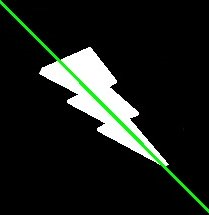# OpenCV-python中的图像处理-轮廓特征

## 目标：

• 查找轮廓的不同特征，如面积，周长，质心，边界框等

## 1. 矩

import numpy as np
import cv2 as cv

ret,thresh = cv.threshold(img,127,255,0)
contours,hierarchy = cv.findContours(thresh, 1, 2)

cnt = contours
M = cv.moments(cnt)
print( M )


cx = int(M['m10']/M['m00'])
cy = int(M['m01']/M['m00'])


## 2. 轮廓面积

area = cv.contourArea(cnt)


## 3. 轮廓周长

perimeter = cv.arcLength(cnt,True)


## 4. 轮廓近似

epsilon = 0.1*cv.arcLength(cnt,True)
approx = cv.approxPolyDP(cnt,epsilon,True)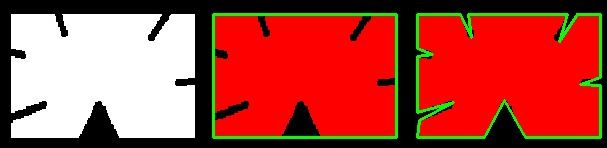## 5. 凸包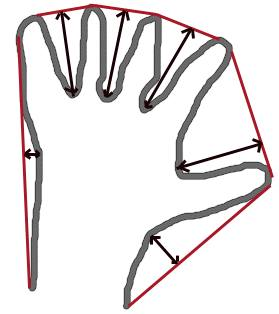hull = cv.convexHull(points[, hull[, clockwise[, returnPoints]]


• points：是我们传入的轮廓。
• hull：是输出，通常我们忽略它。
• clocwise：方向标志。如果为True，则输出凸包顺时针方向。否则，它逆时针方向。
• returnPoints：默认为True。然后它返回凸包点的坐标。如果为False，则返回与凸包点对应的轮廓点的索引。

hull = cv.convexHull(cnt)


## 6. 检查凸性

k = cv.isContourConvex(cnt)


## 7. 边界矩形

7.a.直边矩形

x,y,w,h = cv.boundingRect(cnt)
cv.rectangle(img,(x,y),(x+w,y+h),(0,255,0),2)


7.b.旋转矩形

rect = cv.minAreaRect(cnt)
box = cv.boxPoints(rect)
box = np.int0(box)
cv.drawContours(img,[box],0,(0,0,255),2)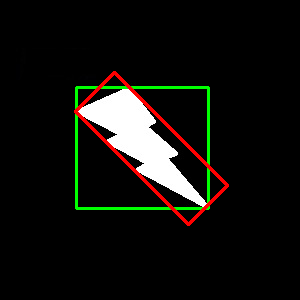## 8. 最小外接圈

(x,y),radius = cv.minEnclosingCircle(cnt)
center = (int(x),int(y))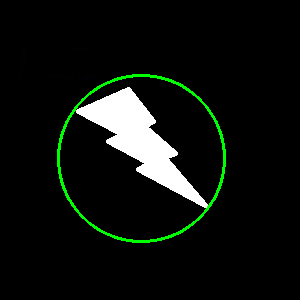## 9. 椭圆拟合

ellipse = cv.fitEllipse(cnt)
cv.ellipse(img,ellipse,(0,255,0),2)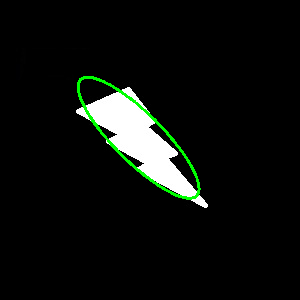## 10. 拟合一条线

rows,cols = img.shape[:2]
[vx,vy,x,y] = cv.fitLine(cnt, cv.DIST_L2,0,0.01,0.01)
lefty = int((-x*vy/vx) + y)
righty = int(((cols-x)*vy/vx)+y)
cv.line(img,(cols-1,righty),(0,lefty),(0,255,0),2)